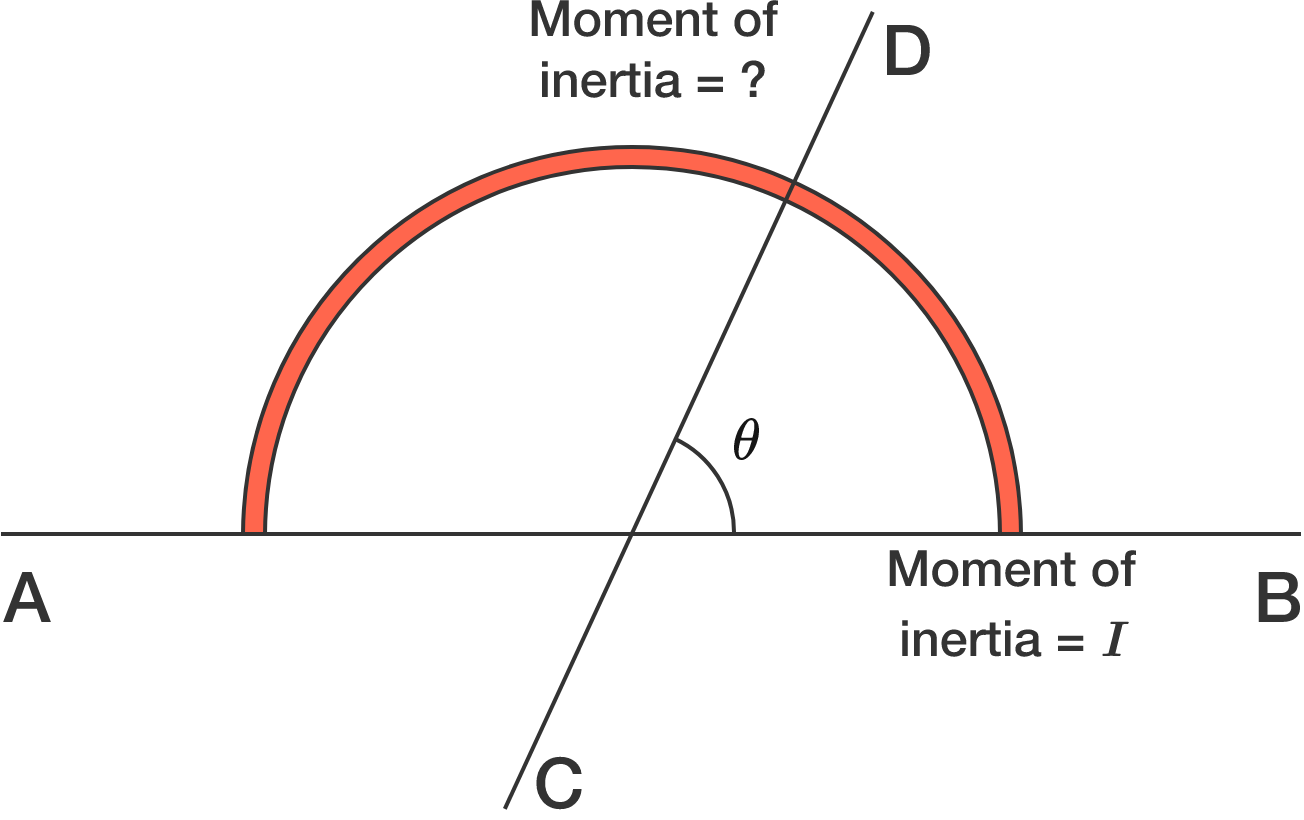# Symmetry of semi circular wire

The moment of inertia of a semi-circular wire about the diameter axis $AB$ is $I.$

What is the moment of inertia about the axis $CD$ passing through the center of the wire?Hint: The moment of inertia depends on the mass and the distribution about the axis.

×